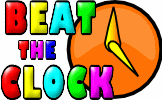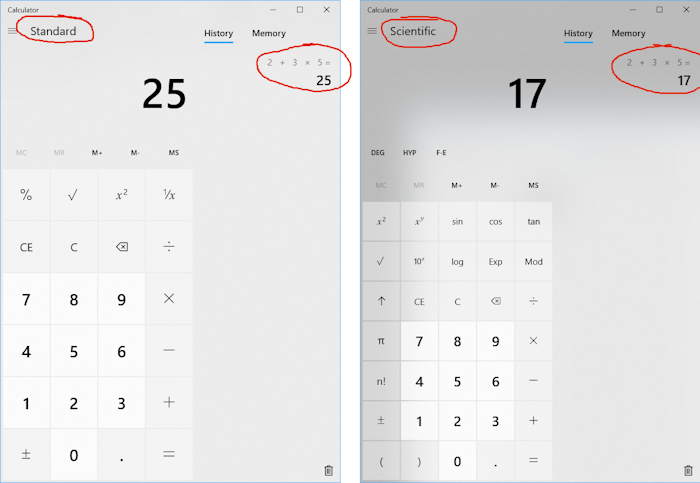# Using a Calculator Level 2

## Questions to test your ability to use a basic calculator, scientific calculator and a graphic display calculator.

##### BIDMASWorkoutBasicScientificGraphic displayDescriptionHelpMore...

Before beginning this exercise you might find it worthwhile to revise the key calculator skills on the Calculator Workout page first.

This is level 2; Using a scientific calculator. The questions provide practice using a calculator to obtain results accurately. You can earn a trophy if you get at least 9 questions correct.

1. Mr Mushnik is 40, his twin brothers are 50 and his twin sisters are 55. What will the sum of their ages be in 17 years time?2.
 6 11
×
 5 63.
 7 13
+
 9 194.
8
 7.3 + 5 2.45.
 − 18.6 −√18.6² − 4 × 5.6 × 5.3 2 × 5.66.
 √6.5² − 1.2² + √3.6² − 1.2² √6.5² − 3.6²7. Calculate 5.4×105 − 3.7×103.8. 1.36 + 1.27 + 1.449. Calculate 1.1 + 1.4π + 43×π×1.53 giving your answer to two decimal places.10. Evaluate 6sin(69o) + cos(15o)² giving your answer to two decimal places.11. If tan(wo) = 0.62 find the value of w to two decimal places.12. Convert the angle 19o42'15" to its decimal equivalent to two decimal places.Check

This is Scientific calculator. You can also try:
BIDMAS Calculator Workout Basic calculator Graphic display calculator

## Instructions

Try your best to answer the questions above. Type your answers into the boxes provided leaving no spaces. As you work through the exercise regularly click the "check" button. If you have any wrong answers, do your best to do corrections but if there is anything you don't understand, please ask your teacher for help.

When you have got all of the questions correct you may want to print out this page and paste it into your exercise book. If you keep your work in an ePortfolio you could take a screen shot of your answers and paste that into your Maths file.

## Transum.org

This web site contains over a thousand free mathematical activities for teachers and pupils. Click here to go to the main page which links to all of the resources available.## More Activities:

Mathematicians are not the people who find Maths easy; they are the people who enjoy how mystifying, puzzling and hard it is. Are you a mathematician?

Comment recorded on the 28 May 'Starter of the Day' page by L Smith, Colwyn Bay:

"An absolutely brilliant resource. Only recently been discovered but is used daily with all my classes. It is particularly useful when things can be saved for further use. Thank you!"

Comment recorded on the 17 November 'Starter of the Day' page by Amy Thay, Coventry:

"Thank you so much for your wonderful site. I have so much material to use in class and inspire me to try something a little different more often. I am going to show my maths department your website and encourage them to use it too. How lovely that you have compiled such a great resource to help teachers and pupils.
Thanks again"

Each month a newsletter is published containing details of the new additions to the Transum website and a new puzzle of the month.

The newsletter is then duplicated as a podcast which is available on the major delivery networks. You can listen to the podcast while you are commuting, exercising or relaxing.

Transum breaking news is available on Twitter @Transum and if that's not enough there is also a Transum Facebook page.

#### Beat The ClockIt is a race against the clock to answer 30 mental arithmetic questions. There are nine levels to choose from to suit pupils of different abilities.

There are answers to this exercise but they are available in this space to teachers, tutors and parents who have logged in to their Transum subscription on this computer.

A Transum subscription unlocks the answers to the online exercises, quizzes and puzzles. It also provides the teacher with access to quality external links on each of the Transum Topic pages and the facility to add to the collection themselves.

Subscribers can manage class lists, lesson plans and assessment data in the Class Admin application and have access to reports of the Transum Trophies earned by class members.

Subscribe

## Go Maths

Learning and understanding Mathematics, at every level, requires learner engagement. Mathematics is not a spectator sport. Sometimes traditional teaching fails to actively involve students. One way to address the problem is through the use of interactive activities and this web site provides many of those. The Go Maths page is an alphabetical list of free activities designed for students in Secondary/High school.

## Maths Map

Are you looking for something specific? An exercise to supplement the topic you are studying at school at the moment perhaps. Navigate using our Maths Map to find exercises, puzzles and Maths lesson starters grouped by topic.

## Teachers

If you found this activity useful don't forget to record it in your scheme of work or learning management system. The short URL, ready to be copied and pasted, is as follows:

Transum,

Thursday, June 13, 2019

"I have just noticed something I hadn’t realised about the Windows calculator. When running in standard mode it operates LTR (2+3x5=25) while in scientific mode it obeys the normal order of operations (2+3x5=17). Worth knowing when learning about BIDMAS or PEMDAS"

Sunday, June 16, 2019

Do you have any comments? It is always useful to receive feedback and helps make this free resource even more useful for those learning Mathematics anywhere in the world. Click here to enter your comments.For All:

## Description of Levels

BIDMAS - Learn about the order of operations.

Calculator Workout - Examples of all the major calculator operations.

CloseLevel 1 - Basic calculator quiz.

Level 2 - Scientific calculator quiz.

Level 3 - Graphic display calculator quiz.

More on this topic including lesson Starters, visual aids and investigations.

Answers to this exercise are available lower down this page when you are logged in to your Transum account. If you don’t yet have a Transum subscription one can be very quickly set up if you are a teacher, tutor or parent.

## Examples

Click on a link below to see an example and an animated key-press diagram of a calculator.

Skill 1: Basic Operations e.g. Calculate the value of $$9+4−7$$

Skill 2: Using Brackets e.g. Calculate the value of $$5(9-4)$$

Skill 3: Percentage of a quantity e.g. What is $$24% of 1800$$?

Skill 4: One quantity as a percentage of another e.g. What is $$45$$ as a percentage of $$150$$?

Skill 5: Squares and square roots e.g. Calculate the value of $$\sqrt{5^2 + 12^2}$$

Skill 6: Indices e.g. $$(3^3 + 2^4)^2$$

Skill 7: Cube roots e.g. Find the value of $$\sqrt{27}$$

Skill 8: Standard Form e.g. Express $$236731$$ in standard form correct to 3 significant figures.

Skill 9: Areas of circles e.g. [Give all answers correct to three significant figures]. e.g. Find the area of a circle with a radius of 9cm.

Skill 10: Fraction calculations e.g. Find the value of $$3\frac13 + 2\frac25$$

Skill 11: Trigonometry e.g. Evaluate $$21 \sin34°$$ to three significant figures 1.

Skill 12: Degrees (or Hours), Minutes and Seconds e.g. Convert the angle 16°35'40" to its decimal equivalent.

Skill 13: Using Variables e.g. If $$A = 1.62$$, $$B = 9.87$$ and $$C = 5.62$$
evaluate $$AB+BC−AC$$.

Skill 14: Combinations e.g. How many different groups of 3 can be selected from 8?

Skill 15: Permutations e.g. In how many different ways could 1st, 2nd and 3rd place prizes can be awarded to 3 of 8 competitors?

Don't wait until you have finished the exercise before you click on the 'Check' button. Click it often as you work through the questions to see if you are answering them correctly. You can double-click the 'Check' button to make it float at the bottom of your screen.

Answers to this exercise are available lower down this page when you are logged in to your Transum account. If you don’t yet have a Transum subscription one can be very quickly set up if you are a teacher, tutor or parent.

Close